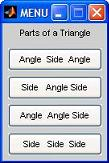# Trigonometry (finding parts of a triangle) - Using Menus

 This program calculates three unknown parts of a triangle when three parts are given (basic trigonometry is used). At least one part given must be the length of a side. We show in this code four possibilities for data entry: Angle, side, angle Side, angle, side Angle, angle, side Side, side, side

Data must be entered in the order it appears in a triangle, either clockwise or counterclockwise.

We are going to start our Matlab coding with a simple menu for the options

opt = menu('Parts of a Triangle', ...
'Angle  Side  Angle',...
'Side   Angle Side',...
'Angle  Angle Side',...
'Side   Side  Side');

This code launches a menu window with four buttons, one for each option according to the problem in our trigonometry.
Note that the first string in the menu is used to write any message that we want at the top. Note also that we can separate long lines of code with the '...' ending.

The value of the option (in this case an integer from 1 to 4) is kept in the 'opt' variable.

This nice window appearsThen, we can define some useful constants

% Define useful constants
c = pi/180;
ea = 'Enter angle (in degrees): ';
es = 'Enter side: ';

The 'c' value is used as a converter from radians to degrees. All trigonometric functions in Matlab work in radians by default.

The last strings are used to ask for information from the keyboard. Instead of writing 'input('Enter angle (in degrees): ')' many times along the code, we can define the string 'ea' and write 'input(ea)', achieving exactly the same result. If we change the string in the definition, we don't have to change all the other lines that contain this string.

Then, we use the 'switch statement' to develop all of the options that solve this problem in trigonometry, case by case.

% Develop all the different cases
switch opt
% Ask for 'angle side angle'
case 1
a(1) = input(ea) * c;
s(3) = input(es);
a(2) = input(ea) * c;
a(3) = pi - a(1) - a(2);
s(1) = s(3) * sin(a(1)) / sin(a(3));
s(2) = s(3) * sin(a(2)) / sin(a(3));
...

If the user choses button 1 in the menu, the code will ask to enter three things from the keyboard, so this is actually an interactive code. It asks for an angle, then for a side, and finally for another angle of the triangle under study. Then, the code solves the problem according to basic trigonometric rules, skips all the other cases and goes to the final of the program, where the results are displayed...

if min(a) < 0
error('Angles of a triangle cannote be less than zero...')
end

% Show results
disp(' ')
disp('Sides...')
s
disp('Opposite angles...')
a = a/c

The complete code, including the four cases, is as follows:

%  Clear memory, clear screen and save empty lines
clc; clear;
format compact

opt = menu('Parts of a Triangle', ...
'Angle  Side  Angle',...
'Side   Angle Side',...
'Angle  Angle Side',...
'Side   Side  Side');

% Define useful constants
c = pi/180;
ea = 'Enter angle (in degrees): ';
es = 'Enter side: ';

% Develop all the different cases
switch opt
% Ask for 'angle side angle'
case 1
a(1) = input(ea) * c;
s(3) = input(es);
a(2) = input(ea) * c;
a(3) = pi - a(1) - a(2);
s(1) = s(3) * sin(a(1)) / sin(a(3));
s(2) = s(3) * sin(a(2)) / sin(a(3));

% Ask for 'side angle side'
case 2
s(3) = input(es);
a(1) = input(ea) * c;
s(2) = input(es);
s(1) = sqrt(s(3)^2+s(2)^2-2*s(3)*s(2)*cos(a(1)));
a(2) = sin(a(1)) * s(2) / s(1);
a(2) = asin(a(2));
a(3) = pi - a(1) - a(2);

% Ask for 'angle angle side'
case 3
a(3) = input(ea) * c;
a(2) = input(ea) * c;
s(3) = input(es);
a(1) = pi - a(2) - a(3);
s(1) = s(3) * sin(a(1)) / sin(a(3));
s(2) = s(3) * sin(a(2)) / sin(a(3));

% Ask for 'side side side'
case 4
s(1) = input(es);
s(2) = input(es);
s(3) = input(es);
a(1) = (s(2)^2 + s(3)^2 - s(1)^2)/(2 * s(2) * s(3));
a(1) = acos(a(1));
a(2) = sin(a(1)) * s(2) / s(1);
a(2) = asin(a(2));
a(3) = pi - a(1) - a(2);
end

if min(a) < 0
error('Angles of a triangle cannote be less than zero...')
end

% Show results
disp(' ')
disp('Sides...')
s
disp('Opposite angles...')
a = a/c

We try it like this: choose the first button on the menu, and answer...

Enter angle (in degrees): 25.7
Enter side: 21.67
Enter angle (in degrees): 33.92

Then, the Matlab response for our trigonometry menu is:

Sides...
s =
10.8931   14.0173   21.6700
Opposite angles...
a =
25.7000   33.9200  120.3800

Note that there is one option missing in the menu. The option of 'side side angle' is not included in the code... Try to develop it yourself. If you can do it then you know that your Matlab knowledge is becoming great!!!

Good luck!

From 'Trigonometry' to home

From 'Trigonometry' to 'Matlab Cookbook'